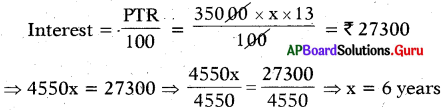SCERT AP 7th Class Maths Solutions Pdf Chapter 7 Ratio and Proportion Ex 7.7 Textbook Exercise Questions and Answers.

## AP State Syllabus 7th Class Maths Solutions 7th Lesson Ratio and Proportion Ex 7.7

Question 1.
Calculate the simple interest accrued on a sum oR 12600, at the rate of 9% per annum for 2 years.
Given principle = ₹ 12600
Time = 2 years
Rate of Interest = 9%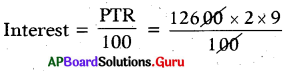∴ Simple Interest = ₹ 2268

Question 2.
Calculate the simple interest accrued for 3 years and the total amount on a sum of ₹ 85000, at the rate of 11% per annum.
Given Principle = ₹ 85000
Time = 3 years
Rate of Interest = 11%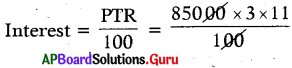Simple Interest = ₹ 28,050
Total amount = Principle + Interest
= 85000 + 28050
∴ Total amount = ₹ 1,13,050Question 3.
In what time will ₹ 45000 amounts to ₹ 63000, If the simple Interest Is calculated 10% per annum?
Method 1:
Given principle = ₹ 45000
Rate of Interest = 10%
Amount = ₹ 63000
Let the time = x years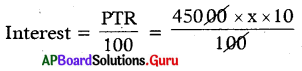Interest = ₹ 4500 x
Amount = Principle + Interest
= 45000 + 4500x = 63000
= 4500x = 63000 – 45000 = 18000
⇒ x = $$\frac{18000}{4500}$$ = 4
∴ Time = 4 years

Method 2:
Given A = ₹ 63000;
P = ₹ 45000; R = 10%; T=?
So I = A – P = 63000 – 45000 = ₹ 18000

But I = $$\frac{\text { PTR }}{100}$$
⇒ 18000 = $$\frac{45000 \times T \times 10}{100}$$
∴ T = $$\frac{18000 \times 100}{45000 \times 10}$$ = 4 years

Question 4.
On a certain amount at the rate of 12% per annum for time 3 years the toti1 interest becomes ₹ 18000. What is the principal amount?
Given Rate of Interest = 12%
Time.= 3 years
Interest = ₹ 18000
Let Principle = ₹ x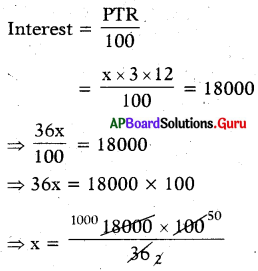∴ Principle = ₹ 50,000Question 5.
In what time the simple interest accrued on a sum of?35000 at the rate of 13% per annum becomes?27300?